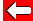# Common Denominators

So how do you find a common denominator? Easy - simply multiply both denominators together. e.g. 3/7 and 4/10:

The common denominator is: 7 × 10 = 70.

An equivalent fraction to 3/7 is 30/70 (we must multiply the numerator by 10 too). The equivalent fraction to 4/10 is 28/70 (we must multiply the numerator by 7).

So 3/7 is larger than 4/10, since 30/70 is bigger than 28/70 (both fractions' equivalents).

A calculator shows this to be true: 3/7 is 0.429 (to 3 decimal places). 4/10 is exactly 0.4 of course.Go back a pageMaths MenuGo to next page